• 我们可以通过findContours函数将二值图像的边缘像素点分成多个轮廓，从而逐个提取目标外部轮廓，内部轮廓有待研究。 Python： import cv2 as cv import numpy as np if __name__=="__main__": img=cv.imread("D:/...
• 文章目录前言一、边缘检测二、轮廓提取1.非边缘检测轮廓提取2.边缘检测和轮廓调节总结 前言 opencv中的边缘检测和轮廓提取都是图像识别的重要部分 提示：以下是本篇文章正文内容，下面案例可供参考 一、边缘检测 ...
文章目录前言一、边缘检测二、轮廓提取1.非边缘检测轮廓提取2.边缘检测和轮廓调节总结

前言
opencv中的边缘检测和轮廓提取都是图像识别的重要部分

提示：以下是本篇文章正文内容，下面案例可供参考
一、边缘检测
Canny边缘检测算子是一个多级边缘检测算法，Canny的目标是找到一个最优的边缘检测算法，其评价标准为：低错误率、高定位性、最小响应。
Canny边缘检测步骤
Canny函数
Python:
edges = cv.Canny( image, threshold1, threshold2[, edges[, apertureSize[, L2gradient]]] )
image 源图像，需是单通道8位图像
edges 输出的边缘图像，需和源图像具有一样的大小和类型
threshold1 第一个滞后性阀值，double
threshold2 第二个滞后性阀值，double
apertureSize 应用Sobel算子孔径大小，默认值：3
L2gradient a flag, indicating whether a more accurate L 2 norm =(dI/dx) 2 +(dI/dy) 2 − − − − − − − − − − − − − − − − √ should be used to calculate the image gradient magnitude ( L2gradient=true ), or whether the default L 1 norm =|dI/dx|+|dI/dy| is enough ( L2gradient=false ).
import cv2 as cv
import numpy as np

def edge_demo(image):
blurred = cv.GaussianBlur(image, (3, 3), 0)  # 高斯模糊
gray = cv.cvtColor(blurred,cv.COLOR_BGR2GRAY)  # 灰路图像
xgrad = cv.Sobel(gray, cv.CV_16SC1, 1, 0)  # xGrodient
ygrad = cv.Sobel(gray, cv.CV_16SC1, 0, 1)  # yGrodient
edge_output = cv.Canny(xgrad, ygrad, 100, 150)  # edge
cv.imshow("Canny Edge",edge_output)

# #  彩色边缘
# dst = cv.bitwise_and(image, image, mask=edge_output)
# cv.imshow("Color Edge", dst)

src=cv.imread("D:\\1111.png")
cv.namedWindow("input image",cv.WINDOW_AUTOSIZE)
cv.imshow("input image",src)
edge_demo(src)
cv.waitKey(0)

cv.destroyAllWindows()


二、轮廓提取
1.非边缘检测轮廓提取
先将图片进行高斯模糊，然后变成灰路图像，再将图片二值化，最后将图片进行轮廓提取。
代码如下（示例）：
这个方法有一个缺点就是自动二值化的阙值会对轮廓提取产生影响，虽然可以自己定义阙值，但是不能每一章照片进行一次调节。
import cv2 as cv
def contours_demo(image):
blurred = cv.GaussianBlur(image, (3, 3), 0)
gray = cv.cvtColor(blurred, cv.COLOR_RGB2GRAY)  # 灰路图像
# ret , binary = cv.threshold(gray, 0, 255,cv.THRESH_BINARY| cv.THRESH_OTSU) # 图像二值化
ret, binary = cv.threshold(gray, 68, 255, cv.THRESH_BINARY )  # 图像二值化
cv.imshow("binary image",binary)

contours, heriachy = cv.findContours(binary,cv.RETR_LIST,cv.CHAIN_APPROX_SIMPLE)
for i, contour in enumerate(contours):
cv.drawContours(image,contours,i,(0,0,255),6)   #6的改为-1可以填充
print(i)
cv.imshow("detect contours", image)

src=cv.imread("D:\\1111.png")
cv.namedWindow("input image",cv.WINDOW_AUTOSIZE)
#cv.imshow("input image",src)
contours_demo(src)
cv.waitKey(0)

cv.destroyAllWindows()

2.边缘检测和轮廓调节
代码如下（示例）：
import cv2 as cv

def edge_demo(image):
blurred = cv.GaussianBlur(image, (3, 3), 0)  # 高斯模糊
gray = cv.cvtColor(blurred,cv.COLOR_BGR2GRAY)  # 灰路图像
xgrad = cv.Sobel(gray, cv.CV_16SC1, 1, 0)  # xGrodient
ygrad = cv.Sobel(gray, cv.CV_16SC1, 0, 1)  # yGrodient
edge_output = cv.Canny(xgrad, ygrad, 100, 150)  # edge
return edge_output
def contours_demo(image):
#  blurred = cv.GaussianBlur(image, (3, 3), 0)
#  gray = cv.cvtColor(blurred, cv.COLOR_RGB2GRAY)  # 灰路图像
# # ret , binary = cv.threshold(gray, 0, 255,cv.THRESH_BINARY| cv.THRESH_OTSU) # 图像二值化
#  ret, binary = cv.threshold(gray, 68, 255, cv.THRESH_BINARY )  # 图像二值化
#  cv.imshow("binary image",binary)

binary = edge_demo(image)

contours, heriachy = cv.findContours(binary,cv.RETR_LIST,cv.CHAIN_APPROX_SIMPLE)
for i, contour in enumerate(contours):
cv.drawContours(image,contours,i,(0,0,255),6)   #6的改为-1可以填充
print(i)
cv.imshow("detect contours", image)

src=cv.imread("D:\\1111.png")
cv.namedWindow("input image",cv.WINDOW_AUTOSIZE)
#cv.imshow("input image",src)
contours_demo(src)
cv.waitKey(0)

cv.destroyAllWindows()

这样就可以避免掉方法一产生的问题，并且还可以很好的实现轮廓的提取

总结
鸣谢
https://blog.csdn.net/weixin_42261213/article/details/93990410
https://blog.csdn.net/miao0967020148/article/details/88623631


展开全文opencv 计算机视觉
• 在上一篇文章Opencv基于Python图像轮廓——轮廓绘制及其特征中，我们已经学习到了轮廓的一些特征，如质心、面积、周长等。在本篇文章，我们将继续学习到以下内容： 提取一些常用的物体属性，如坚实度，等效直径，...
轮廓属性目标1. 常见的轮廓属性1.1 长宽比1.2 范围1.3 坚实度1.4 等效直径1.5 方向1.6 掩码和像素点1.7 最大值，最小值及其位置1.8 平均颜色或平均强度1.9 极端点2. 更多属性2.1 凸性缺陷2.2 点多边形测试2.3 形状匹配
目标
在上一篇文章Opencv基于Python图像轮廓——轮廓绘制及其特征中，我们已经学习到了轮廓的一些特征，如质心、面积、周长等。在本篇文章，我们将继续学习到以下内容：

提取一些常用的物体属性，如坚实度，等效直径，掩模图像，平均强度等
图像轮廓的凸性缺陷以及如何找到它们
查找点到多边形的最短距离
匹配不同的形状

1. 常见的轮廓属性
1.1 长宽比
它是图象边界矩形的宽度与高度的比值。
$Aspect \; Ratio = \frac{Width}{Height}$
以下代码结果是基于上篇文章Opencv基于Python图像轮廓——轮廓绘制及其特征得到的。
# 宽高比
x, y, w, h = cv.boundingRect(cnt)
aspect_ratio = float(w)/h
print(aspect_ratio)


0.891156462585034

1.2 范围
范围是轮廓区域面积与边界矩形区域面积的比值。
$Extent = \frac{Object \; Area}{Bounding \; Rectangle \; Area}$
# 轮廓面积与边界矩形面积的比
area = cv.contourArea(cnt)
x, y, w, h = cv.boundingRect(cnt)
rect_area = w*h
extent = float(area)/rect_area
print(extent)


0.25502414706340554

1.3 坚实度
坚实度是轮廓线面积与其凸包面积之比。
$Solidity = \frac{Contour \; Area}{Convex \; Hull \; Area}$
# 轮廓面积与凸包面积的比
area = cv.contourArea(cnt)
hull = cv.convexHull(cnt)
hull_area = cv.contourArea(hull)
solidity = float(area)/hull_area
print(solidity)


0.9522055259331071

1.4 等效直径
等效直径是面积与轮廓面积相等的圆的直径。
$Equivalent \; Diameter = \sqrt{\frac{4 \times Contour \; Area}{\pi}}$
# 与轮廓面积相等的圆形直径
area = cv.contourArea(cnt)
equi_diameter = np.sqrt(4*area/np.pi)
print(equi_diameter)


79.07515035834193

1.5 方向
取向是物体指向的角度。以下方法还给出了主轴和副轴的长度。
# 方向
(x, y), (MA, ma), angle = cv.fitEllipse(cnt)
print((x, y), (MA, ma), angle)


(269.22100830078125, 255.53665161132812) (34.14046859741211, 225.88348388671875) 139.3023223876953

1.6 掩码和像素点
在某些情况下，我们可能需要构成该对象的所有点，可以按照以下步骤完成：
# 轮廓的掩膜与像素点
img = cv.imread('./data/boundingRect.png', 0)
mask = np.zeros(img.shape, np.uint8)
mask = cv.drawContours(mask, [cnt], 0, 255, -1)
cv.imshow('mask', mask)
cv.waitKey(0)
cv.destroyAllWindows()
pixelpoints = np.transpose(np.nonzero(mask))
#pixelpoints = cv.findNonZero(mask)这里提供了两个方法，一个使用Numpy函数，另一个使用OpenCV函数(最后的注释行)。结果也是一样的，只是略有不同。Numpy给出的坐标是 (行、列)格式，而OpenCV给出的坐标是(x,y)格式。所以基本上结果是可以互换的。注意， row = x, column = y。
1.7 最大值，最小值及其位置
我们可以使用掩码图像找到这些参数。
# 最大值和最小值及其位置
min_val, max_val, min_loc, max_loc = cv.minMaxLoc(img, mask=mask)
print(min_val, max_val, min_loc, max_loc)


255.0 255.0 (225, 183) (225, 183)

1.8 平均颜色或平均强度
在这里，我们可以找到对象的平均颜色，或者可以是灰度模式下物体的平均强度，我们再次使用相同的掩码进行此操作。
# 平均颜色及平均灰度
mean_val = cv.mean(img, mask=mask)
print(mean_val)


(255.0, 0.0, 0.0, 0.0)

1.9 极端点
极点是指对象的最顶部，最底部，最右侧和最左侧的点。
# 极端点
leftmost = tuple(cnt[cnt[:, :, 0].argmin()])
rightmost = tuple(cnt[cnt[:, :, 0].argmax()])
topmost = tuple(cnt[cnt[:, :, 1].argmin()])
bottommost = tuple(cnt[cnt[:, :, 1].argmax()])
print (leftmost, rightmost, topmost, bottommost)


(204, 202) (334, 307) (225, 183) (312, 329)

2. 更多属性
2.1 凸性缺陷
我们已经在Opencv基于Python图像轮廓——轮廓绘制及其特征中学习到关于轮廓的凸包。从这个凸包上的任何偏差都可以被认为是凸性缺陷。OpenCV有一个函数来找到这个，cv.convexityDefects()。一个基本的函数调用如下:
hull = cv.convexHull(cnt,returnPoints = False)
defects = cv.convexityDefects(cnt,hull)


注意： 我们必须在发现凸包时，传递 returnPoints= False ，以找到凸性缺陷。

它返回一个数组，其中每行包含这些值——[起点、终点、最远点、到最远点的近似距离]。我们可以用图像把它形象化。我们画一条连接起点和终点的线，然后在最远处画一个圆。记住，返回的前三个值是cnt的索引。所以我们必须从cnt中获取这些值。
import numpy as np
import cv2 as cv

img = cv.imread('./data/convexityDefects.png')
imggray = cv.cvtColor(img, cv.COLOR_BGR2GRAY)
ret, thresh = cv.threshold(imggray, 127, 255, cv.THRESH_OTSU)
img2, contours, hierarchy = cv.findContours(thresh, cv.RETR_TREE, cv.CHAIN_APPROX_SIMPLE)
cnt = contours
hull = cv.convexHull(cnt, returnPoints=False)
defects = cv.convexityDefects(cnt, hull)
for i in range(defects.shape):
s, e, f, d = defects[i, 0]
start = tuple(cnt[s])
end = tuple(cnt[e])
far = tuple(cnt[f])
cv.line(img, start, end, [0, 255, 0], 3)
cv.circle(img, far, 5, [0, 0, 255], -1)
cv.imshow('convexityDefects', img)
cv.waitKey()
cv.destroyAllWindows()2.2 点多边形测试
这个函数找出图像中一点到轮廓线的最短距离。它返回的距离，点在轮廓线外时为负，点在轮廓线内时为正，点在轮廓线上时为零。
例如，我们可以检查点 (50,50) 如下:
dist = cv.pointPolygonTest(cnt,(50,50),True)

在函数中，第三个参数是measureDist。如果它是真的，它会找到有符号的距离。如果为假，则查找该点是在轮廓线内部还是外部(分别返回+1、-1和0)。
# 点多边形测试
dist = cv.pointPolygonTest(cnt, (50, 50), True)
print(dist)


-202.71408436514716

注意: 如果你不想找到距离，请确保第三个参数为False，因为这是一个耗时的过程。因此，将其设置为False可使速度提高2-3倍。

2.3 形状匹配
OpenCV附带一个函数cv.matchShapes()，该函数使我们能够比较两个形状或两个轮廓，并返回一个显示相似性的度量，结果越低，匹配越好，它是根据矩值计算出来的。
import cv2 as cv
import numpy as np
img1 = cv.imread('./data/convexityDefects.png', 0)
img2 = cv.imread('./data/matchShapes.png', 0)
ret, thresh = cv.threshold(img1, 127, 255, 0)
ret, thresh2 = cv.threshold(img2, 127, 255, 0)
img2, contours, hierarchy = cv.findContours(thresh, cv.RETR_TREE, cv.CHAIN_APPROX_SIMPLE)
cnt1 = contours
img2, contours, hierarchy = cv.findContours(thresh2, cv.RETR_TREE, cv.CHAIN_APPROX_SIMPLE)
cnt2 = contours
ret = cv.matchShapes(cnt1, cnt2, 1, 0.0)
print(ret)


1.1241123297334此匹配结果值较高，说明了匹配效果较差


展开全文opencv 计算机视觉
• python opencv检测并提取目标颜色 【Python+OpenCV】识别颜色方块并提取轮廓 OpenCV颜色提取——获取图片上某一区域的颜色平均HSV值——颜色识别
python opencv检测并提取目标颜色
【Python+OpenCV】识别颜色方块并提取轮廓
OpenCV颜色提取——获取图片上某一区域的颜色平均HSV值——颜色识别
图像的腐蚀(erode)和膨胀(dilate) 开运算以及闭运算------理论知识及其对应函数


展开全文• 当通过阈值分割提取到图像中的目标物体后，就需要通过边缘检测来提取目标物体的轮廓，使用这两种方法基本能够确定物体的边缘或者前景。接下来，通常需要做的是拟合这些边缘的前景，如拟合出包含前景或者边缘像素点的...
1，轮廓发现
当通过阈值分割提取到图像中的目标物体后，就需要通过边缘检测来提取目标物体的轮廓，使用这两种方法基本能够确定物体的边缘或者前景。接下来，通常需要做的是拟合这些边缘的前景，如拟合出包含前景或者边缘像素点的最小外包矩形、圆、凸包等几何形状，为计算它们的面积或者模板匹配等操作打下坚实的基础。
一个轮廓代表一系列的点（像素），这一系列的点构成一个有序的点集，所以可以把一个轮廓理解为一个有序的点集。
轮廓发现是基于图像边缘提取的基础，寻找对象轮廓的方法，所以边缘提取的阈值选定会影响最终轮廓的发现。
2，对象测量
opencv 中轮廓特征包括：如面积，周长，质心，边界框等。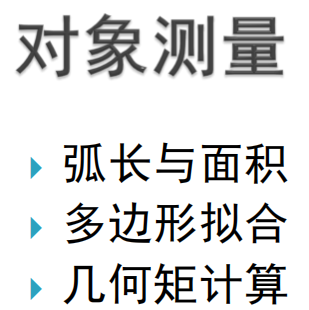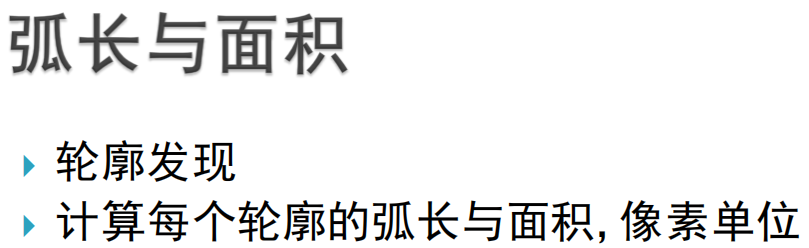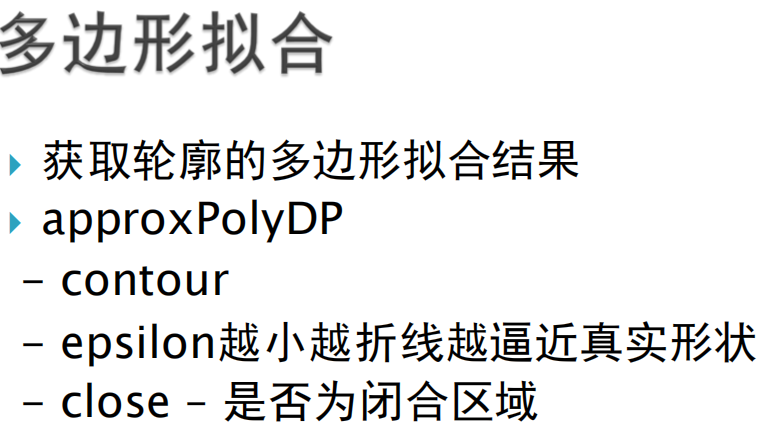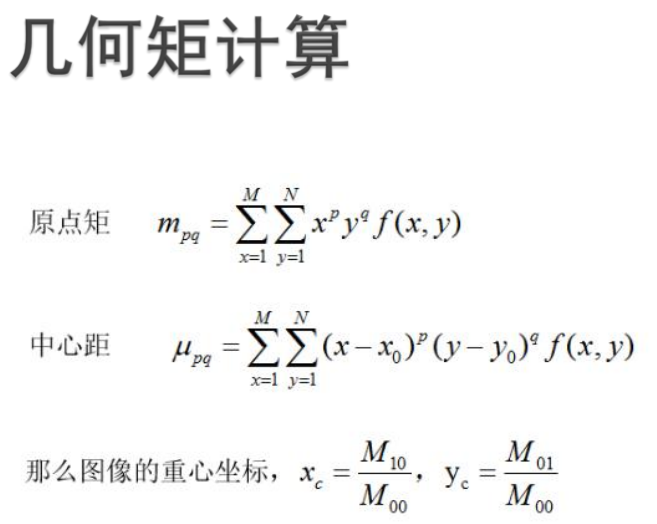3，轮廓发现源代码：
import cv2 as cv
import numpy as np

def edge_demo(image):
blurred = cv.GaussianBlur(image, (9, 9), 5)
gray = cv.cvtColor(blurred, cv.COLOR_BGR2GRAY)
# X Gradient
xgrad = cv.Sobel(gray, cv.CV_16SC1, 1, 0)
# Y Gradient
ygrad = cv.Sobel(gray, cv.CV_16SC1, 0, 1)
#edge
#edge_output = cv.Canny(xgrad, ygrad, 50, 150)
edge_output = cv.Canny(gray, 30, 100)
cv.imshow("Canny Edge", edge_output)
return edge_output

def contours_demo(image):
dst = cv.GaussianBlur(image, (3, 3), 0)
gray = cv.cvtColor(dst, cv.COLOR_BGR2GRAY)
ret, binary = cv.threshold(gray, 0, 255, cv.THRESH_BINARY | cv.THRESH_OTSU)
cv.imshow("binary image", binary)#直接二值化的图像
#binary = edge_demo(image)   #过边缘处理后的图像
#可以是直接二值化的图像也可以是经过边缘处理后的图像（两种方法视情况而定）

#cloneImage, contours, heriachy = cv.findContours(binary, cv.RETR_EXTERNAL, cv.CHAIN_APPROX_SIMPLE)
contours, hierarchy = cv.findContours(binary,cv.RETR_TREE,cv.CHAIN_APPROX_SIMPLE)
cv.drawContours(image,contours,-1,(0,0,255),3)
cv.imshow("detect contours", image)

src = cv.imread("F:/images/coin.png")
cv.namedWindow("input image", cv.WINDOW_AUTOSIZE)
cv.imshow("input image", src)
contours_demo(src)
cv.waitKey(0)

cv.destroyAllWindows()


运行结果：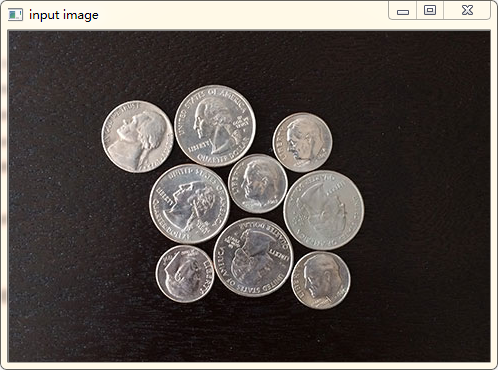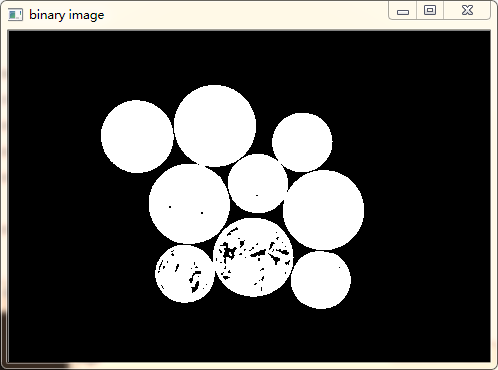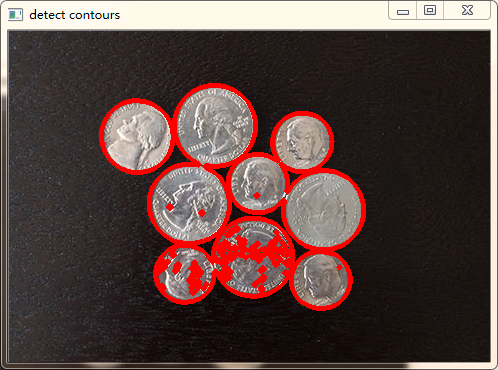注意：对于cv.findContours：opencv2返回两个值：contours：hierarchy。注:opencv3会返回三个值,分别是img, countours, hierarchy
4，对象测量源代码：
import cv2 as cv
import numpy as np

def measure_object(image):
gray = cv.cvtColor(image, cv.COLOR_BGR2GRAY)
ret, binary = cv.threshold(gray, 0, 255, cv.THRESH_BINARY | cv.THRESH_OTSU)
print("threshold value : %s"%ret)
cv.imshow("binary image", binary)
dst = cv.cvtColor(binary, cv.COLOR_GRAY2BGR)
contours, hierarchy = cv.findContours(binary,cv.RETR_EXTERNAL,cv.CHAIN_APPROX_SIMPLE)
for i, contour in enumerate(contours):
area = cv.contourArea(contour)  #轮廓的面积
x, y, w, h = cv.boundingRect(contour) #轮廓的外接矩形
rate = min(w, h)/max(w, h)#轮廓外接矩形的宽高比
print("rectangle rate : %s"%rate)
mm = cv.moments(contour)#求轮廓的几何矩
#print(type(mm))#字典型数据
cx = mm['m10']/mm['m00']#原点的零阶矩
cy = mm['m01']/mm['m00']
cv.circle(dst, (np.int(cx), np.int(cy)), 3, (0, 0, 255), -1)##画出中心点，-1表示填充
#cv.rectangle(dst, (x, y), (x+w, y+h), (0, 0, 255), 2)#绘制轮廓的外接矩形
print("contour area %s"%area)
approxCurve = cv.approxPolyDP(contour,4,True) #多边形逼近 4是与阈值的间隔大小，越小越易找出，True是是否找闭合图像
"""
cv.contourArea(contour)      #获取每个轮廓面积
cv.boundingRect(contour)     #获取轮廓的外接矩形
cv.moments(contour)          #求取轮廓的几何距
cv.arcLength(contour,True)　　#求取轮廓的周长，指定闭合
approxPolyDP(curve, epsilon, closed, approxCurve=None)
第一个参数curve：输入的点集，直接使用轮廓点集contour
第二个参数epsilon：指定的精度，也即是原始曲线与近似曲线之间的最大距离。
第三个参数closed：若为true,则说明近似曲线是闭合的，反之，若为false，则断开。
第四个参数approxCurve：输出的点集，当前点集是能最小包容指定点集的。画出来即是一个多边形；

print(approxCurve)　　#打印每个轮廓的特征点
print(approxCurve.shape)　　#打印该点集的shape，第一个数是代表了点的个数，也就是边长连接逼近数
"""
print(approxCurve.shape)
if approxCurve.shape > 6:
cv.drawContours(dst, contours, i, (0, 255, 0), 2)
if approxCurve.shape == 4:
cv.drawContours(dst, contours, i, (0, 0, 255), 2)
if approxCurve.shape == 3:
cv.drawContours(dst, contours, i, (255, 0, 0), 2)
cv.imshow("measure-contours", dst)
cv.imshow("measure-contours", dst)

src = cv.imread("F:/images/contours.png")
cv.namedWindow("input image", cv.WINDOW_AUTOSIZE)
cv.imshow("input image", src)
measure_object(src)
cv.waitKey(0)

cv.destroyAllWindows()


运行结果：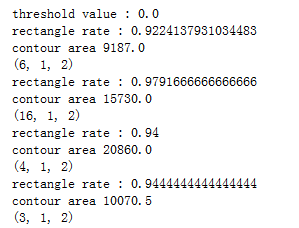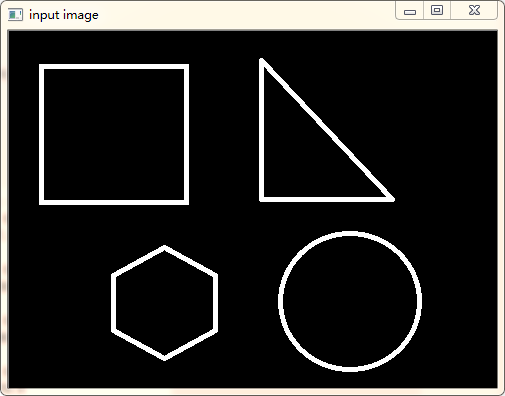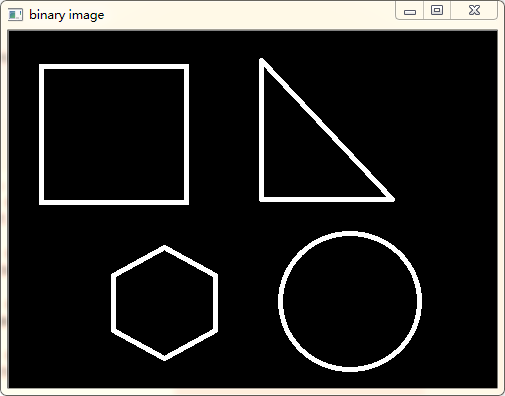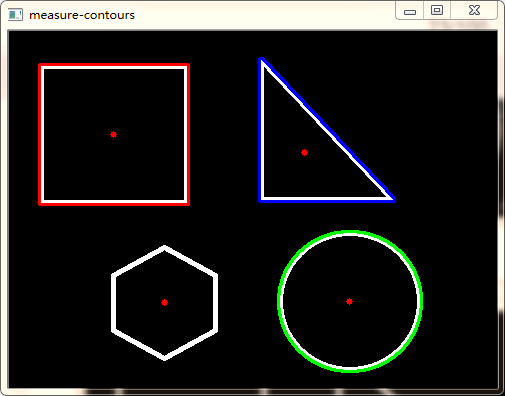展开全文• 对于一般的图像提取轮廓，介绍了一个很好的方法，但是对于有噪声的图像，并不能很好地捕获到目标物体。 比如对于我的鼠标，提取轮廓效果并不好，因为噪声很多： 所以本文增加了去掉噪声的部分。 首先加载原始图像...
• 在图像的处理中有时候需要对图像中的目标区域提出出轮廓 读取图像 调用OpenCV的库使用cv.imread()来读取图像。 图像为 灰度化 二值化 提取轮廓时，图像一般都是二值化后的图像。在本次程序中选用cv2.THRESH_...opencv 图像识别 计算机视觉
• ## opencv-python轮廓属性

千次阅读 2020-04-21 21:56:41
目标： 学习提取一些常用的对象属性，如Solidity，Equivalent Diameter，Mask image，Mean Intensity 1. Aspect Ratio（长宽比） 它是对象的边界矩形的宽度与高度的比。 Aspect Ratio=WidthHeight Aspect\ ...opencv 计算机视觉 opengl numpy unity
• 对于一般的图像提取轮廓，这篇博文介绍了一个很好的方法，但是对于有噪声的图像，并不能很好地捕获到目标物体。 　比如对于我的鼠标，提取轮廓效果并不好，因为噪声很多： 　所以本文增加了去掉噪声的部分。 ...
• 下面小编就为大家带来一篇python-opencv在有噪音的情况下提取图像的轮廓实例。小编觉得挺不错的，现在就分享给大家，也给大家做个参考。一起跟随小编过来看看吧 对于一般的图像提取轮廓，介绍了一个很好的方法，但是...opencv image
• 找出轮廓并画出轮廓 import cv2 import numpy as np image = cv2.imread("D:/2.jpg") gray = cv2.cvtColor(image,cv2.COLOR_BGR2GRAY) ret,thresh =cv2.threshold(gray,50,255,cv2.THRESH_BINARY) cv2.imshow(...
• 提取目标区域并标注前言一、blob分析二、有关代码函数的注释与用法1、cv2.threshold（）阈值处理2、cv2.findContours（）轮廓检测3、cv2.boundingRect(c)矩形边框4、cv2.moments()图像的矩5、cv2.circle（）画圆心6...opencv 计算机视觉
• 目标 在这里，我们将学习提取一些常用的物体属性，如坚实度，等效直径，掩模图像，平均强度等。更多的功能可以在Matlab regionprops文档中找到。 (注:质心、面积、周长等也属于这一类，但我们在上一章已经见过) 1. ...
• 使用cv2.findContours函数查找轮廓时需要传入一个轮廓提取模式的参数（Contour_Retrieval_Mode），通常设置为cv2.RETR_LIST 和 cv2.RETR_TREE。代表什么意思？ 结果会得到三个数组，第一个是图像，第二个是轮廓，第...opencv
• 但是这些都是针对指节区域提取，或者针对旋转位置纠正，或者针对手指轮廓提取，并没有将这些东西整合起来。今天这篇就记录一下提取的流程。（仅仅适用于我的目标图片） 先看看效果：（尺寸都经过拉伸了） 流程：...
• 当我们通过阈值分割提取到图像中的目标物体后，我们就需要通过边缘检测来提取目标物体的轮廓，使用这两种方法基本能够确定物体的边缘或者前景。接下来，我们通常需要做的是拟合这些边缘的前景，如拟合出包含前景或者...
• 目标 查找轮廓的不同特征，例如面积，周长，重心，边界框等，这些特征在未来的图像识别中，会大量的用到。 矩的概念 图像识别的一个核心问题是图像的特征提取，简单描述即为用一组简单的数据（图像描述量）来描述...opencv
• 目标 查找轮廓的不同特征，例如面积，周长，重心，边界框等，这些特征在未来的图像识别中，会大量的用到。 矩的概念 图像识别的一个核心问题是图像的特征提取，简单描述即为用一组简单的数据（图像描述...opencv
• 一 简介 目标检测即为在图像中找到自己感兴趣的部分，将其分割出来进行下一步操作，可避免背景的干扰。以下介绍几种基于opencv的单目标检测算法，算法总体思想先尽量将目标区域的像素值...5 提取轮廓并找出目标外接矩形
• 目标： 1.通过使用opencv获得不同物体的轮廓，以及轮廓所对应的特征，比如说面积、周长、质心、边界框等等。 2.学习一些与轮廓提取有关的函数。
• 目标： 学习提取一些常用的对象属性，如Solidity，Equivalent Diameter，Mask image，Mean Intensity 1. Aspect Ratio（长宽比） 它是对象的边界矩形的宽度与高度的比。 Aspect&amp;amp;nbsp;Ratio=...
• 在前面的内容中我们使用函数 cv2.findContours来查找轮廓，我们需要传入一个参数：轮廓提取模式（Contour_Retrieval_Mode）。我们总是把它设置为 cv2.RETR_LIST 或者是 cv2.RETR_TREE，效果还可以。但是它们到底代表...
• 轮廓发现：当通过阈值分割提取到图像中的目标物体后，就需要通过边缘检测来提取目标物体的轮廓，使用这两种方法基本能够确定物体的边缘或者前景。接下来，通常需要做的是拟合这些边缘的前景，如拟合出包含前景或者...opencv 计算机视觉 图像识别# python目标轮廓提取python 订阅